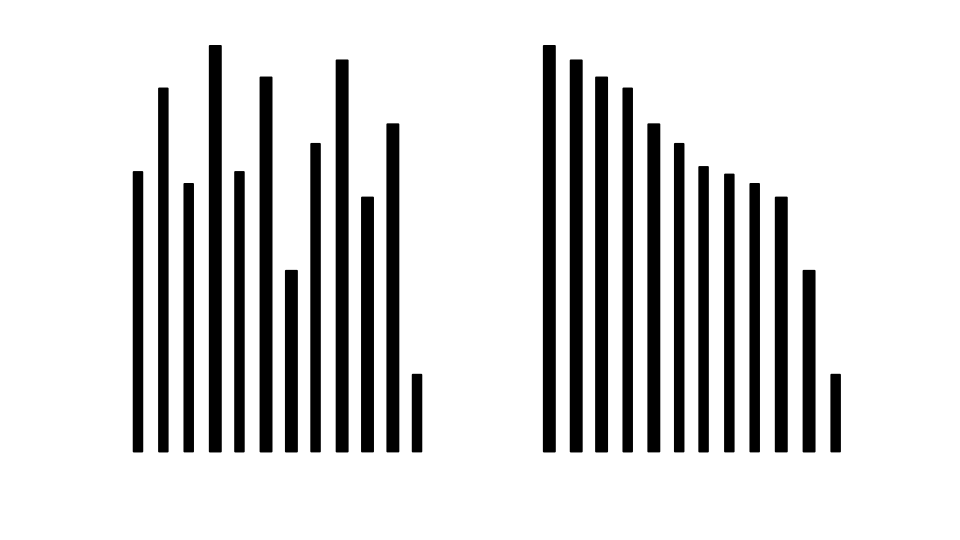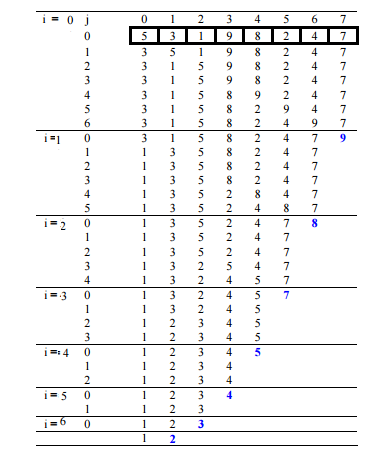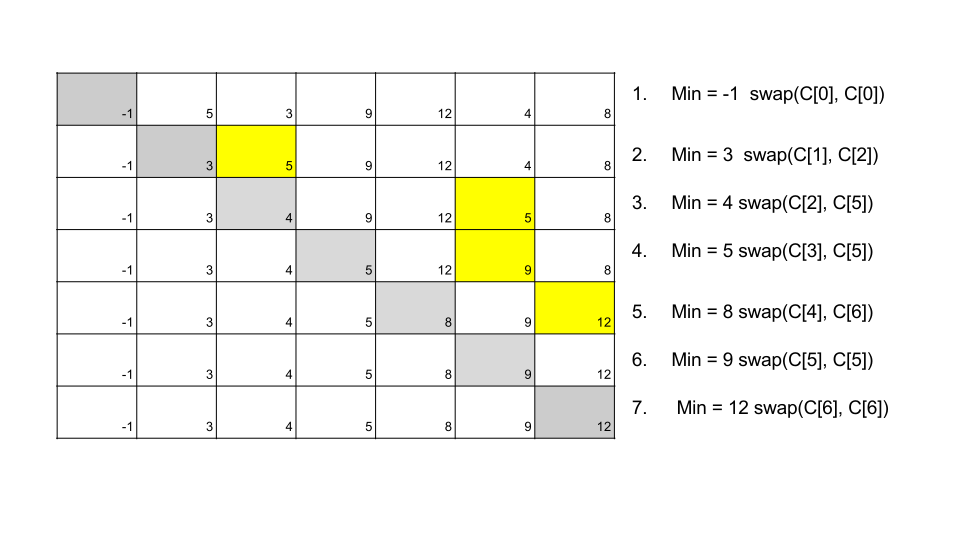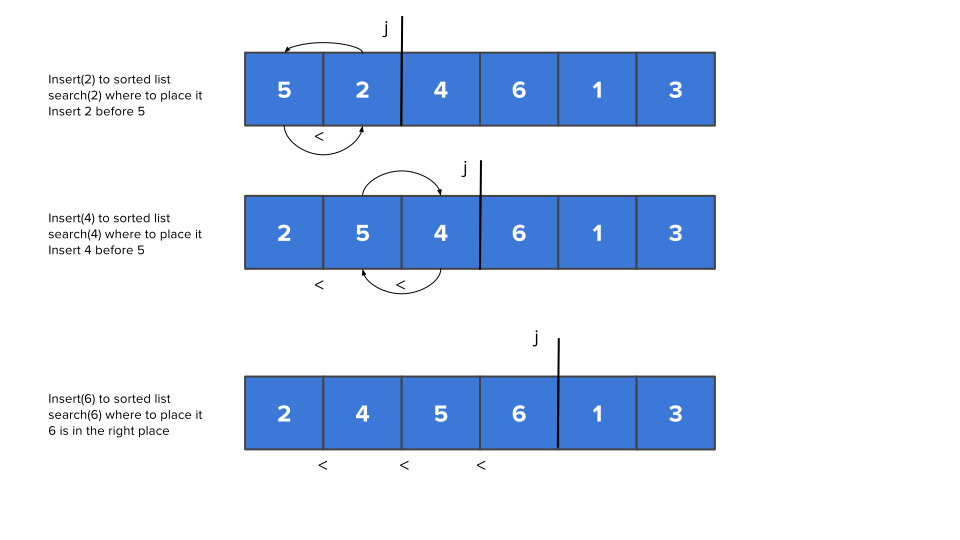# Sorting

## Introduction

• Sorting is the process of assigning the elements of a collection (e.g. list) in a particular order (e.g. put words in an alphabetical order).

• A simplistic systematic way to sort the elements of a collection is, first, to compare two values from the collection seeing which is the smaller (or greater).

• And, second, if the two values are not in the correct order, based on their comparison, we need to exchange them.

• This exchange is expensive and, therefore, the total number of the needed exchanges will indicate the efficiency of a sorting algorithm.

## The Sorting Problem

• Input: Sequence of n values $$c_1,c_2,c_3,...,c_n$$
• Output: Reordered (permutation) $$c'_1,c'_2,c'_3,...,c'_n$$ of the input sequence with the order $$c'_1 \leq c'_2 \leq c'_3 ... \leq c'_n$$

Example:

• Input: $$6,4,3,1,2,5$$
• Output: $$1,2,3,4,5,6$$

## The Sorting ProblemYou have to arrange the sticks in descending order, how would you process this?

## Bubble Sort

A simple sort algorithm where list elements “bubbles” to the position in the list where it belongs (e.g holding the order invariant)

• How does it work?
• Compares each pair of elements in a list
• Swap the order of the pair of elements, if they are in the wrong order
• Repeatedly traverse the list and swap pair of elements until the entire list is ordered

## Bubble Sort: Overview

Given a list $$C[]$$ with $$n$$ elements

1. Compare $$C$$ with $$C$$
• if $$C > C$$, swap the elements
• if $$C < C$$, elements are in order
2. Traverse to the next element, $$C$$ and compare it with $$C$$
• if $$C > C$$, swap the elements
• if $$C < C$$, elements are in order
• Do this for every pair of elements until the end of the list.
3. Repeatedly perform steps 1 and 2 $$n$$ times

## Bubble Sort: Example

Sort the following list $$C = [7,6,1]$$

This would require 2 comparisons for a list of 3 values

First iteration

1. Compare $$7$$ with $$6$$, swap them $$[7,6,1]$$ -> $$[\textbf{6,7},1]$$
2. Compare $$7$$ with $$1$$, swap them $$[6,7,1]$$ -> $$[6,\textbf{1,7}]$$

Second iteration

1. Compare $$6$$ with $$1$$, swap them $$[6,1,7]$$ -> $$[\textbf{1,6},7]$$

Third iteration

Values are in order

## Bubble Sort: Exercise

Sort the following list $$C = [5,3,1,9,8,2,4,7]$$ using bubble sort

## Bubble Sort: Solution## Bubble Sort: Implementation

def bubble_sort(list):
n_times = len(list)
# Traverse all list elements
for i in range(n_times):
print "round: " + str(i) + " with " + str(list)
for j in range(0, n_times-i-1):
# traverse the list from 0 to n-i-1 (e.g number of possible swap left)
print str(list[j]) + " > " + str(list[j+1]) + "?"
if list[j] > list[j+1] :
list[j], list[j+1] = list[j+1], list[j]
print "swap"
else:
print "no swap"
return list

## Bubble Sort: Complexity

• How many comparison does each loop perform? (inner loop)
• For each element in the list: $$n - 1$$ comparisons
• bubble sort does $$O(n)$$ comparisons
• How many times does it need to order the list? (outer loop)
• The list contains $$n$$ elements and has $$O(n)$$ elements

For each iteration of loop variable $$i$$, there are $$n-i$$ comparisons performed. Thus, Bubble sort conducts $$O(n)$$ operations on an $$O(n)$$ number of elements that leads to a time complexity of $$O(n^2)$$

## Bubble Sort: Complexity (2)

• When $$i = 1$$, no comparisons
• When $$i = 2$$, one comparison is performed
• When $$i = 3$$, two comparisons are performed and so forth

Therefore, when $$i = n$$, $$n - 1$$ comparisons are performed irrespective of the input

For a list with $$n$$ elements does:

$$1 + 2 + 3 + 4 + \cdots + (n - 2) + (n - 1) = O(n^2)$$ comparisons

Last, the space complexity is $$O(1)$$ (e.g constant amount of memory)

## Selection Sort

• A natural sorting algorithm in which we first find minimum element in the list, then find the second minimum, third minimum and so forth and put them in an increasing order
• Similar to bubble sort, for $$i^{th}$$ iteration in the loop, performs $$n - i$$ comparisons
• Performs a linear search to find $$i^{th}$$ minimum element

## Selection Sort: Overview

key idea: Search and swap algorithm

1. Find smallest element
2. Swap it with the first element in the list
3. Repeat the steps above with the second, third position in the list until it is sorted

## Selection Sort: Example

Sort the following list $$C = [-1,5,3,9,12,4,8]$$## Selection Sort: Implementation

def selectionSort(list):
for i in range(len(list)-1):
print "index " + str(i) + " in the list"
min = i
for j in range(i + 1, len(list)):
if list[j] < list[min]:
min = j
print "min value is " + str(list[min]) + " at index " + str(min)
list[i], list[min] = list[min], list[i]
return list


## Selection Sort: Complexity

The selection sort makes the same number of comparisons as the bubble sort and is therefore also $$O(n^2)$$. This is due to the linear search for finding the minimum element in which we have to pass $$i - n$$ values

However, due to the reduction in the number of exchanges, the selection sort typically executes faster than the bubble sort.

What is the best case?

## Insertion Sort

A simple sorting algorithm that works in similar fashion to sorting cards with our hands

• The design is of an incremental algorithm; it constructs the sorted list one number at a time.
• Compared to the previous algorithms; during the $$i^{th}$$ iteration, the first $$i - 1$$ elements (named $$S$$) are sorted and the $$i^{th}$$ element is inserted in $$S$$ by first making a linear search on $$S$$ and placing it in the right position.
• Useful for sorting smaller lists

## Insertion Sort: Example